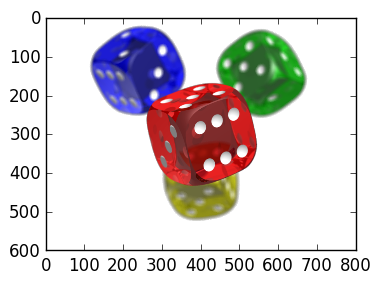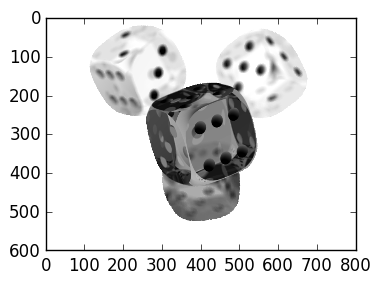# Lecture 21: Images¶

In this lecture we consider manipulating images in Julia. Before that, we wrap up some loose ends on stability:

## Back substition is backward stable¶

Using similar logic to the dot product case from last lecture, the following theorem can be proven, showing that back substitution is backward stable:

Theorem Approximating the problem $f(U) = U^{-1} \mathbf b$ by backsubstition $\tilde f(U) = {\rm backsubstitution(U,\mathbf b)$ in floating point arithmetic satisfies

$\tilde f(U) = f(U + \Delta U)$

where the relative backward error satisfies

$${\|\Delta U\|_\infty \over \|U\|_\infty} \leq {n \epsilon \over 1 -n\epsilon}.$$

A trivial consequence is that the forward error is small provided that the $\infty$-condition number

$$\kappa_\infty(U) \triangleq \|U\|_\infty \|U^{-1}\|_\infty$$

is small:

Corollary

$${\|f(U) - f(\tilde U)\|_\infty \over \|f(U)\|_\infty} \leq \kappa_\infty(U) {n \epsilon \over 1-n\epsilon}$$

We omit the precise statement, but we also have that QR with Given's rotations is backward stable.

## PLU is not stable¶

We finally come to a surprise: the PLU decomposition is not stable! We can demonstrate this on a very simple example:

$$A = \begin{pmatrix} 1 & && & 1 \cr -1 & 1 && & 1\cr -1 & -1 & 1 && 1 \cr \vdots & \vdots & \vdots & \ddots & \vdots \cr -1 & -1 & -1 & \cdots & 1 \end{pmatrix}$$

This matrix is well-conditioned:

In :
n=100

A=2eye(n)-tril(ones(n,n))
A[1:n-1,end]=ones(n-1)

cond(A)

Out:
44.80225124630286

The QR Decomposition, because it is stable, preserves this condition number:

In :
Q,R=qr(A)

cond(Q),cond(R)

Out:
(1.0000000000000018,44.80225124630287)

The PLU Decomposition, on the other hand, has very badly conditioned components (in this case $P=I$:

In :
L,U,p=lu(A)

cond(L),cond(U)

Out:
(9.345713008686627e17,8.451004001521529e29)

This bad conditioning translates into very inaccurate solution, even for the inbuilt \ command. This compares unfavourably with the QR Decomposition, which is perfectly accurate:

In :
b=rand(n)
x_backslash=A\b
x_QR=(R\(Q'*b))
x_LU=U\(L\b)
x_inv=inv(A)*b

norm(x_inv-x_QR),norm(x_LU-x_inv)

Out:
(4.943922429291265e-15,9.685147727311062e6)

We can check the error in residual: that is, see how well the approximation satisfies $Ax=b$:

In :
norm(A*x_inv-b),norm(A*x_LU-b)

Out:
(9.27040380578984e-15,7.746521550447148e7)

Perturbing the matrix $A$ by a small amount causes the PLU decomposition to become stable:

In :
n=100

A=2eye(n)-tril(ones(n,n))
A[1:n-1,end]=ones(n-1)

A=A+0.0001*randn(n,n)

Q,R=qr(A)
L,U,p=lu(A)

cond(A),cond(L),cond(U)

Out:
(44.80474788446134,210.96228170781936,68.99809165265951)

This is a big open problem: explaining why with high probability that PLU Decomposition is stable. This is the reason \ uses PLU: the chance of failure is small, and PLU is roughly 2x as fast as QR.

# Images in Julia¶

We now consider image analysis. We can load an image in Julia using PyPlot:

In :
using PyPlot

imshow(img);This is a 600 x 800 pixel image. Each pixel has 4 channels: the red, green, blue and alpha component. This is stored by a 600 x 800 x 4 tensor:

In :
size(img)

Out:
(600,800,4)

Each value is between 0. and 1.:

In :
maximum(img),minimum(img)

Out:
(1.0f0,0.0f0)

A tensor is like a matrix just with one more index. For example:

In :
A=rand(3)     # random vector of length 3
A=rand(3,3)   # random matrix of size 3 x 3
A=rand(3,3,3) # random matrix of size 3 x 3 x 3

Out:
3x3x3 Array{Float64,3}:
[:, :, 1] =
0.225026  0.679698   0.875816
0.161986  0.0402596  0.269381
0.515684  0.640174   0.654053

[:, :, 2] =
0.175512  0.0302496  0.329362
0.893919  0.262348   0.400141
0.720663  0.82769    0.650222

[:, :, 3] =
0.353372  0.81447    0.0399681
0.079338  0.390212   0.735976
0.668979  0.0419518  0.502528 

The entries are accessed using three components:

In :
A[1,3,2]

Out:
0.3293624516851563

Just like matrices, tensors are actually stored as a single vector in memory:

In :
vec(A)

Out:
27-element Array{Float64,1}:
0.225026
0.161986
0.515684
0.679698
0.0402596
0.640174
0.875816
0.269381
0.654053
0.175512
0.893919
0.720663
0.0302496
⋮
0.329362
0.400141
0.650222
0.353372
0.079338
0.668979
0.81447
0.390212
0.0419518
0.0399681
0.735976
0.502528 

We can access the R,G,B and A components by creating 600 x 800 matrices follows:

In :
R=img[:,:,1]
G=img[:,:,2]
B=img[:,:,3]
A=img[:,:,3]

size(R)

Out:
(600,800)

We now create a grey-scale image, by removing the A component and setting the R,G and B components to the same values. We also swap white and black to make it more visible:

In :
myimg=zeros(size(img,1),size(img,2),3)

myimg[:,:,1]=1-R
myimg[:,:,2]=1-R
myimg[:,:,3]=1-R

grey=myimg[:,:,1]

imshow(myimg)Out:
PyObject <matplotlib.image.AxesImage object at 0x3152d4e50>
In [ ]: